Поиск даташита (1.687.043 компонентов) Где искатьв наименованиив описании### Datasheet: J309 (Fairchild Semiconductor)

N-Channel RF Amplifier
Скачать:PDFZIP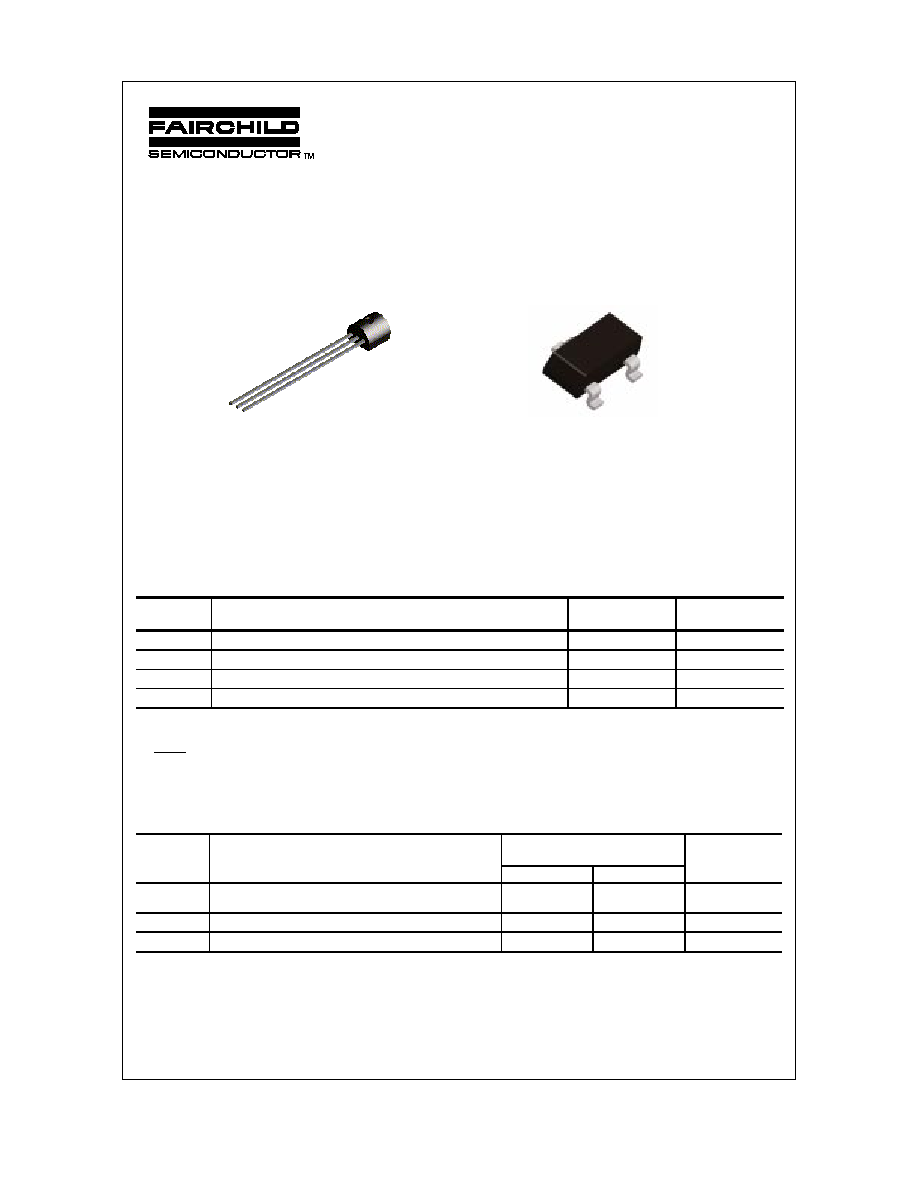J309 / J310 / MMBFJ309 / MMBFJ310
Discrete POWER & Signal
Technologies
J309
J310
N-Channel RF Amplifier
This device is designed for VHF/UHF amplifier, oscillator and mixer
applications. As a common gate amplifier, 16 dB at 100 MHz and
12 dB at 450 MHz can be realized. Sourced from Process 92.
Absolute Maximum Ratings*
TA = 25�C unless otherwise noted
*
These ratings are limiting values above which the serviceability of any semiconductor device may be impaired.
NOTES:
1) These ratings are based on a maximum junction temperature of 150 degrees C.
2) These are steady state limits. The factory should be consulted on applications involving pulsed or low duty cycle operations.
Thermal Characteristics
TA = 25�C unless otherwise noted
MMBFJ309
MMBFJ310
Symbol
Parameter
Value
Units
V
DS
Drain-Source Voltage
25
V
V
GS
Gate-Source Voltage
- 25
V
I
GF
Forward Gate Current
10
mA
T
J
,T
stg
Operating and Storage Junction Temperature Range
-55 to +150
C
Symbol
Characteristic
Max
Units
J309 / J310
*MMBFJ309
P
D
Total Device Dissipation
Derate above 25
C
350
2.8
225
1.8
mW
mW/
C
R
JC
Thermal Resistance, Junction to Case
125
C/W
R
JA
Thermal Resistance, Junction to Ambient
357
556
C/W
SOT-23
Mark: 6U / 6T
G
S
D
G
S
D
TO-92
*
Device mounted on FR-4 PCB 1.6" X 1.6" X 0.06."
1997 Fairchild Semiconductor Corporation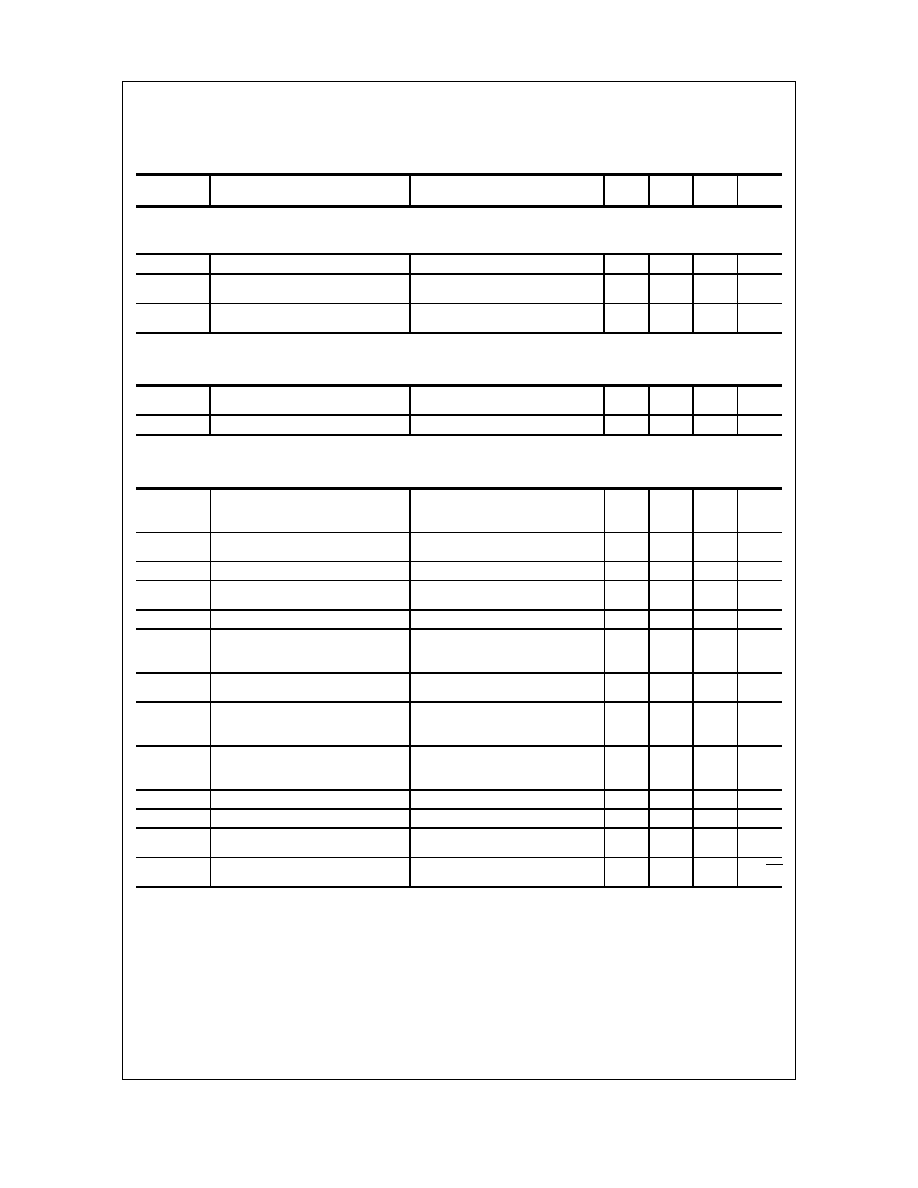J309 / J310 / MMBFJ309 / MMBFJ310
Electrical Characteristics
TA = 25�C unless otherwise noted
OFF CHARACTERISTICS
Symbol
Parameter
Test Conditions
Min
Typ
Max Units
V
(BR)GSS
Gate-Source Breakdown Voltage
I
G
= - 1.0
A, V
DS
= 0
- 25
V
I
GSS
Gate Reverse Current
V
GS
= - 15 V, V
DS
= 0
V
GS
= - 15 V, V
DS
= 0, T
A
= 125
C
- 1.0
- 1.0
nA
A
V
GS(off)
Gate-Source Cutoff Voltage
V
DS
= 10 V, I
D
= 1.0 nA
J309
J310
- 1.0
- 2.0
- 4.0
- 6.5
V
V
ON CHARACTERISTICS
I
DSS
Zero-Gate Voltage Drain Current*
V
DS
= 10 V, V
GS
= 0
J309
J310
12
24
30
60
mA
mA
V
GS(
f
)
Gate-Source Forward Voltage
V
DS
= 0, I
G
= 1.0 mA
1.0
V
SMALL SIGNAL CHARACTERISTICS
*
Pulse Test: Pulse Width
300
m
s, Duty Cycle
2.0%
Re
(
y
is)
Common-Source Input Conductance
V
DS
= 10, I
D
= 10 mA, f = 100 MHz
J309
J310
0.7
0.5
mmhos
mmhos
Re
(
y
os)
Common-Source Output
Conductance
V
DS
= 10, I
D
= 10 mA, f = 100 MHz
0.25
mmhos
G
pg
Common-Gate Power Gain
V
DS
= 10, I
D
= 10 mA, f = 100 MHz
16
dB
Re
(
y
fs)
Common-Source Forward
Transconductance
V
DS
= 10, I
D
= 10 mA, f = 100 MHz
12
mmhos
Re
(
y
ig)
Common-Gate Input Conductance
V
DS
= 10, I
D
= 10 mA, f = 100 MHz
12
mmhos
g
fs
Common-Source Forward
Transconductance
V
DS
= 10, I
D
= 10 mA, f = 1.0 kHz
J309
J310
10,000
8000
20,000
18,000
mhos
mhos
g
os
Common-Source Output
Conductance
V
DS
= 10, I
D
= 10 mA, f = 1.0 kHz
150
mhos
g
fg
Common-Gate Forward Conductance V
DS
= 10, I
D
= 10 mA, f = 1.0 kHz
J309
J310
13,000
12,000
mhos
mhos
g
og
Common-Gate Output Conductance
V
DS
= 10, I
D
= 10 mA, f = 1.0 kHz
J309
J310
100
150
mhos
mhos
C
dg
Drain-Gate Capacitance
V
DS
= 0, V
GS
= - 10, f = 1.0 MHz
2.0
2.5
pF
C
sg
Source-Gate Capacitance
V
DS
= 0, V
GS
= - 10, f = 1.0 MHz
4.1
5.0
pF
NF
Noise Figure
V
DS
= 10 V, I
D
= 10 mA,
f = 450 MHz
3.0
dB
e
n
Equivalent Short-Circuit Input
Noise Voltage
V
DS
= 10 V, I
D
= 10 mA,
f = 100 Hz
6.0
nV/
Hz
N-Channel RF Amplifier
(continued)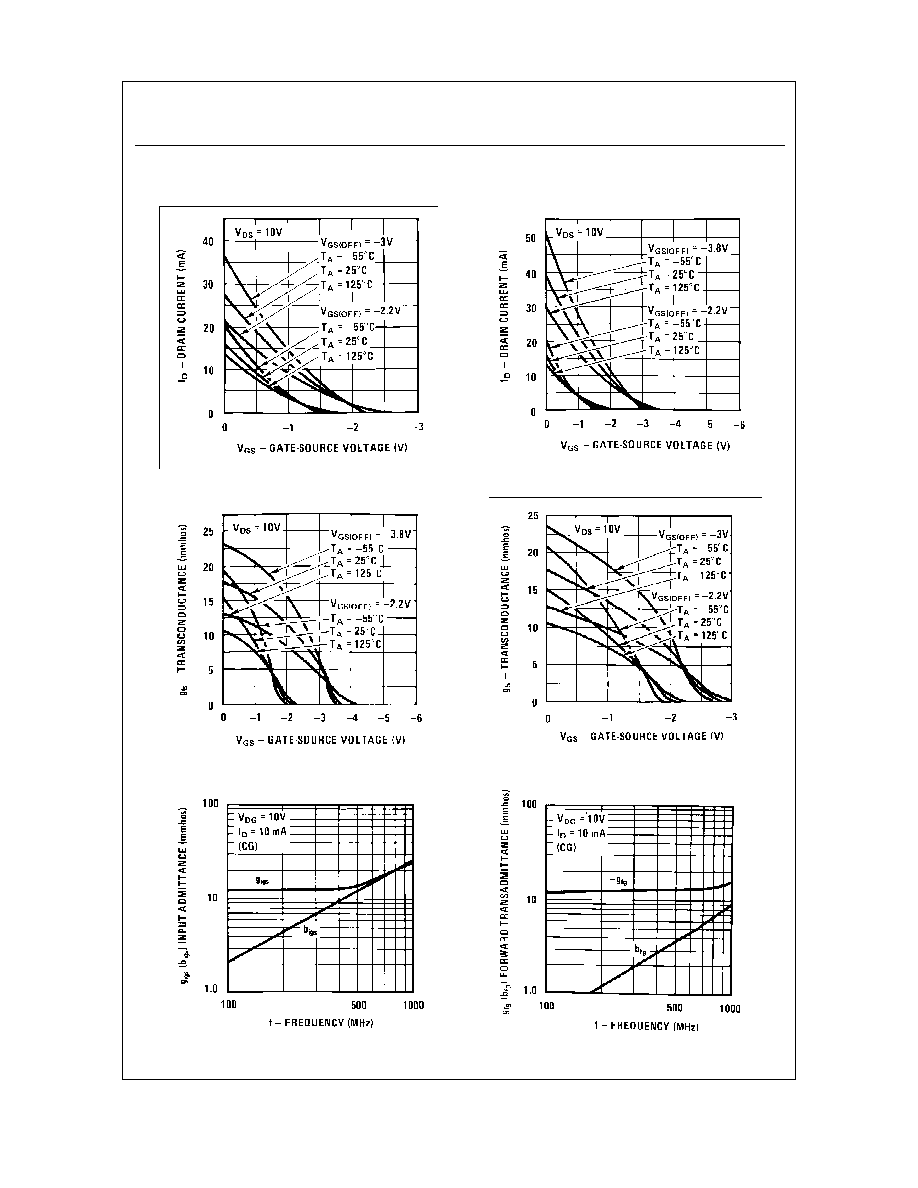J309 / J310 / MMBFJ309 / MMBFJ310
Typical Characteristics
Transfer Characteristics
Transfer Characteristics
Transfer Characteristics
N-Channel RF Amplifier
(continued)
Transfer Characteristics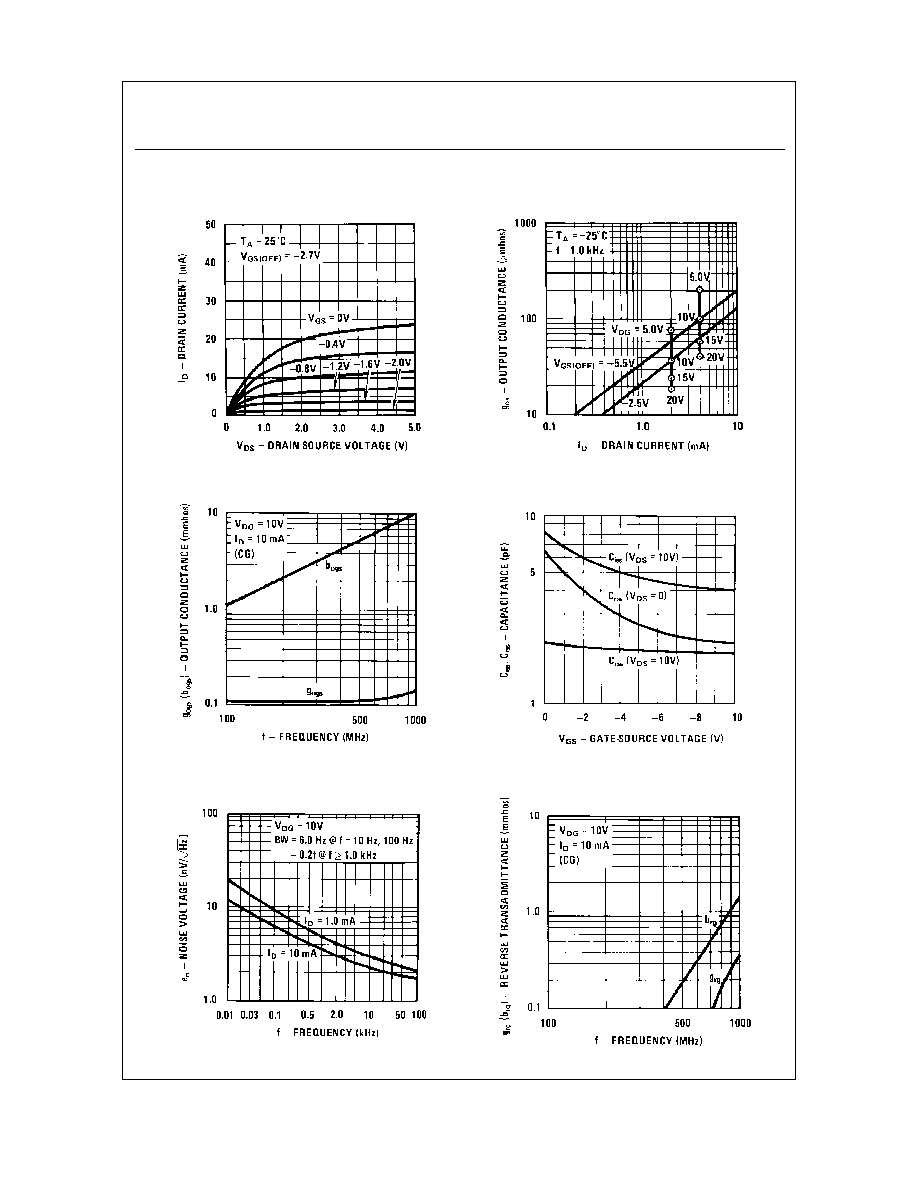J309 / J310 / MMBFJ309 / MMBFJ310
Typical Characteristics
(continued)
Capacitance vs. Voltage
Output Conductance vs.
Drain Current
Common Drain-Source
Noise Voltage vs. Frequency
N-Channel RF Amplifier
(continued)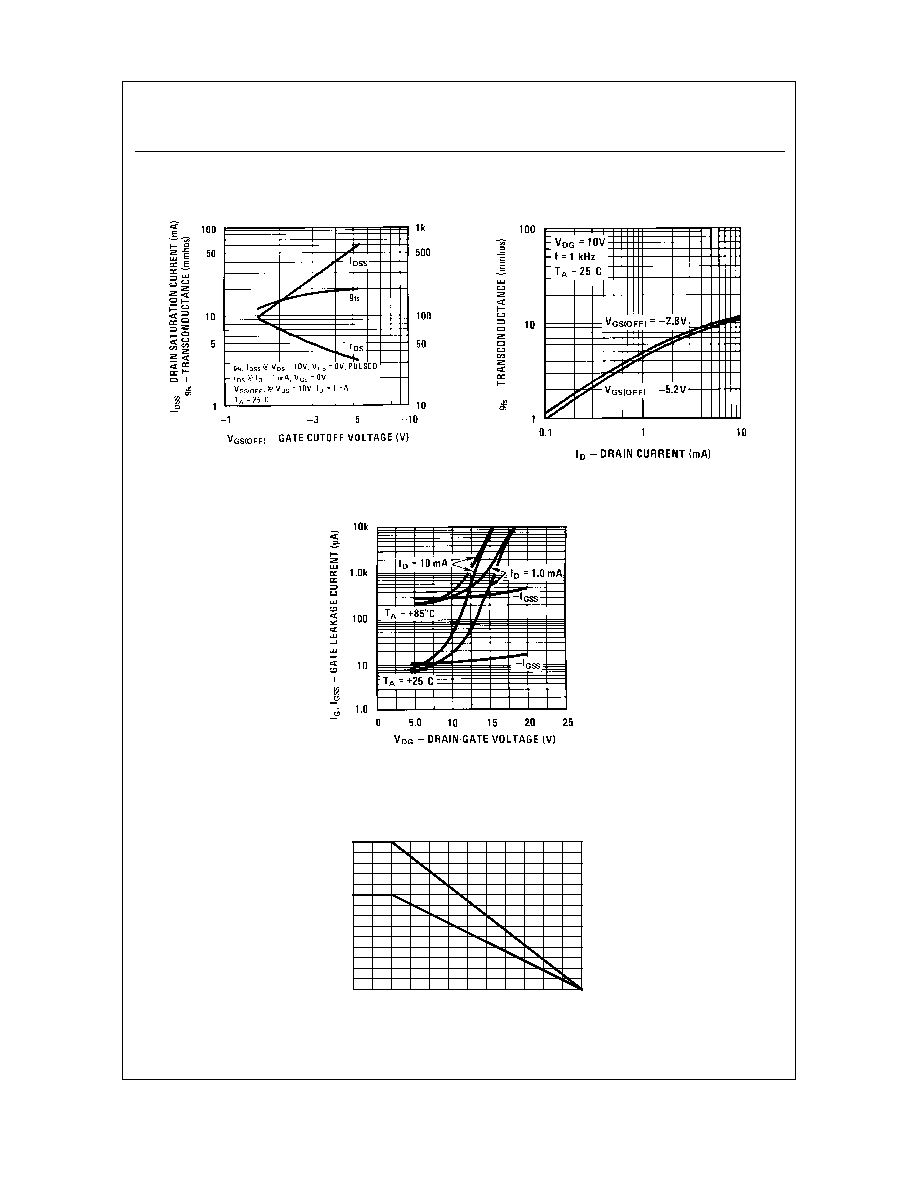J309 / J310 / MMBFJ309 / MMBFJ310
N-Channel RF Amplifier
(continued)
Typical Characteristics
(continued)
Transconductance vs.
Drain Current
Parameter Interactions
Leakage Current vs. Voltage
POWER DISSIPATION vs
AMBIENT TEMPERATURE
0
25
50
75
100
125
150
0
50
100
150
200
250
300
350
TEMPERATURE ( C)
P
- P
O
W
E
R DIS
S
I
P
A
T
I
O
N (m
W
)
TO-92
SOT-23© 2020 • ChipFind• Контакты• Главная страница